# 【机器学习】贝叶斯网络

## 2. 细数贝叶斯网络

### 2.2 贝叶斯学派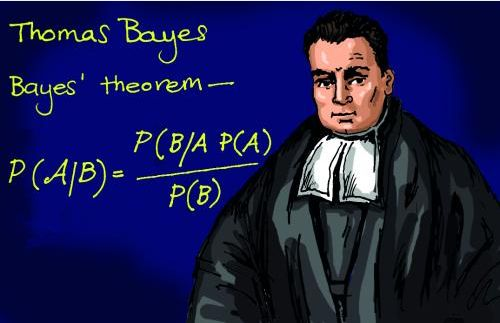• 频率派把需要推断的参数θ看做是固定的未知常数，即概率虽然是未知的，但最起码是确定的一个值，同时，样本X 是随机的，所以频率派重点研究样本空间，大部分的概率计算都是针对样本X 的分布；
• 而贝叶斯派的观点则截然相反，他们认为参数是随机变量，而样本X 是固定的，由于样本是固定的，所以他们重点研究的是参数的分布。

### 2.3 贝叶斯定理$$P(A|B)=\frac{P(A\cap_{}B)}{P(B)}$$

### 2.4 贝叶斯网络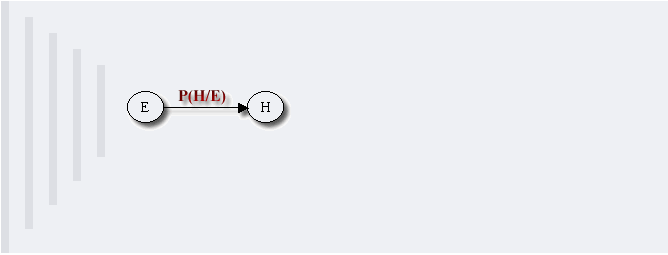$$P(x_1,…,x_k)=P(x_k|x_1,…,x_{k-1})…P(x_2|x_1)P(x_1)$$

#### 2.4.1 贝叶斯网络的结构形式

1. head-to-head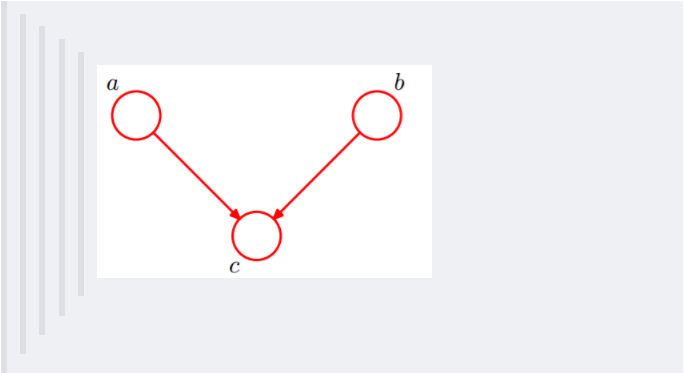2. tail-to-tail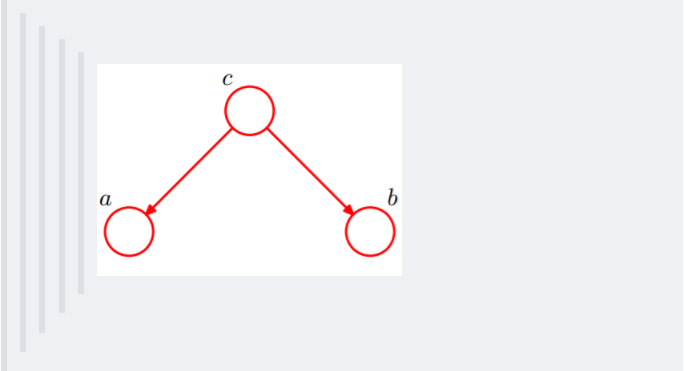1. 在c未知的时候，有：P(a,b,c)=P(c)P(a|c)P(b|c)，此时，没法得出P(a,b) = P(a)P(b)，即c未知时，a、b不独立。
2. 在c已知的时候，有：P(a,b|c)=P(a,b,c)/P(c)，然后将P(a,b,c)=P(c)P(a|c)P(b|c)带入式子中，得到：P(a,b|c)=P(a,b,c)/P(c) = P(c)P(a|c)P(b|c) / P(c) = P(a|c)*P(b|c)，即c已知时，a、b独立。

3. head-to-tail1. c未知时，有：P(a,b,c)=P(a)P(c|a)P(b|c)，但无法推出P(a,b) = P(a)P(b)，即c未知时，a、b不独立。

2. c已知时，有：P(a,b|c)=P(a,b,c)/P(c)，且根据P(a,c) = P(a)P(c|a) = P(c)P(a|c)，可化简得到：

$$P(a,b|c)$$

=$$P(a,b,c)/P(c)$$

=$$P(a)P(c|a)P(b|c) / P(c)$$

=$$P(a,c)*P(b|c)/P(c)$$

=$$P(a|c)*P(b|c)$$

所以，在c给定的条件下，a，b被阻断(blocked)，是独立的，称之为head-to-tail条件独立。

这个head-to-tail其实就是一个链式网络，如下图所示：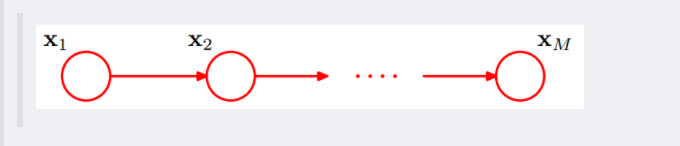根据之前对head-to-tail的讲解，我们已经知道，在xi给定的条件下，xi+1的分布和x1,x2…xi-1条件独立。意味着啥呢？意味着：xi+1的分布状态只和xi有关，和其他变量条件独立。通俗点说，当前状态只跟上一状态有关，跟上上或上上之前的状态无关。这种顺次演变的随机过程，就叫做马尔科夫链（Markov chain）。对于马尔科夫链我们下一节再细讲。

#### 2.4.2 因子图

wikipedia上是这样定义因子图的：将一个具有多变量的全局函数因子分解，得到几个局部函数的乘积，以此为基础得到的一个双向图叫做因子图（Factor Graph）。

$$g(x_1,x_2,x_3,x_4,x_5)=f_A(x_1)f_B(x_2)f_C(x1,x2,x3)f_D(x_3,x_4)f_E(x_3,x_5)$$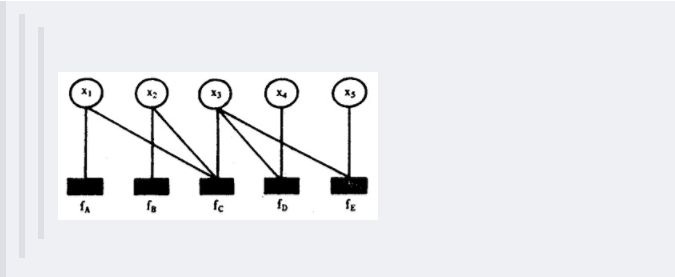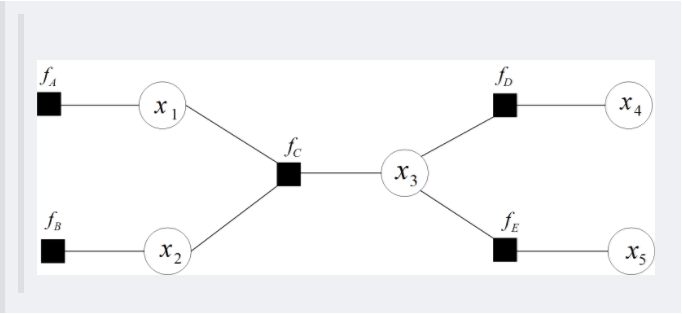### 2.5 朴素贝叶斯

• 一个特征出现的概率与其他特征（条件）独立；
• 每个特征同等重要。

$$P(A|B)=\frac{P(B|A)P(A)}{P(B)}=P(类别|特征)=\frac{P(特征|类别)P(类别)}{P(特征)}$$$$P(嫁|不帅,性格不好,矮,不上进)=\frac{P(不帅,性格不好,矮,不上进|嫁)P(嫁)}{P(不帅,性格不好,矮,不上进)}$$

1. 我们这么想，假如没有这个假设，那么我们对右边这些概率的估计其实是不可做的，这么说，我们这个例子有4个特征，其中帅包括{帅，不帅}，性格包括{不好，好，爆好}，身高包括{高，矮，中}，上进包括{不上进，上进}，那么四个特征的联合概率分布总共是4维空间，总个数为233*2=36个。

36个，计算机扫描统计还可以，但是现实生活中，往往有非常多的特征，每一个特征的取值也是非常之多，那么通过统计来估计后面概率的值，变得几乎不可做，这也是为什么需要假设特征之间独立的原因。

2. 假如我们没有假设特征之间相互独立，那么我们统计的时候，就需要在整个特征空间中去找，比如统计p(不帅、性格不好、身高矮、不上进|嫁),我们就需要在嫁的条件下，去找四种特征全满足分别是不帅，性格不好，身高矮，不上进的人的个数，这样的话，由于数据的稀疏性，很容易统计到0的情况。 这样是不合适的。

• 算法逻辑简单,易于实现（算法思路很简单，只要使用贝叶斯公式转化即可！）
• 分类过程中时空开销小（假设特征相互独立，只会涉及到二维存储）

## 3. 基于贝叶斯的一些问题

1. 解释朴素贝叶斯算法里面的先验概率、似然估计和边际似然估计？
• 先验概率：就是因变量（二分法）在数据集中的比例。这是在你没有任何进一步的信息的时候，是对分类能做出的最接近的猜测。
• 似然估计：似然估计是在其他一些变量的给定的情况下，一个观测值被分类为1的概率。例如，“FREE”这个词在以前的垃圾邮件使用的概率就是似然估计。
• 边际似然估计：边际似然估计就是，“FREE”这个词在任何消息中使用的概率。

## 4. 生成式模型和判别式模型的区别

• 判别模型(discriminative model)通过求解条件概率分布P(y|x)或者直接计算y的值来预测y。

线性回归（Linear Regression）,逻辑回归（Logistic Regression）,支持向量机（SVM）, 传统神经网络（Traditional Neural Networks）,线性判别分析（Linear Discriminative Analysis），条件随机场（Conditional Random Field）

• 生成模型（generative model）通过对观测值和标注数据计算联合概率分布P(x,y)来达到判定估算y的目的。

朴素贝叶斯（Naive Bayes）, 隐马尔科夫模型（HMM）,贝叶斯网络（Bayesian Networks）和隐含狄利克雷分布（Latent Dirichlet Allocation）、混合高斯模型